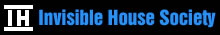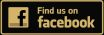# Creating the One System for the Practicus GradeThe instruction includes one of three fundamental keys to the Practicus that will, in a technical way, be used in the creation of the fifth elemental weapon. Aid in the accomplishment of the exercises or verification of all end results can be obtained by writing to the Invisible House Society.

Let the Practicus place a large diagram of the Tree of Life upon the wall, with the sephiroth and paths numbered from 1-32, and with no other attributions or markings.

Let six triangles be identified from the Tree of Life diagram, and let each triangle be separately drawn, with the numbers and appropriate tarot attribution.

Let five of these triangles be properly identified and each labeled with one of the formula L–L–L–L–L (Light – Life – Love – Liberty – Law).

Let two squares be identified from the Tree of Life diagram, and let each square be separately drawn, with the numbers and appropriate tarot attribution.

Let one cube be identified from the Tree of Life diagram, and let the cube be separately drawn, with the numbers and appropriate tarot attribution.

Let one upright pentagram be identified from the Tree of Life diagram, and let the pentagram be separately drawn, with the numbers and appropriate tarot attribution.

Let one adverse pentagram be identified from the Tree of Life diagram, and let the pentagram be separately drawn, with the numbers and appropriate tarot attribution.

Let two pentagons be identified from the Tree of Life diagram, and let each pentagon be separately drawn, with the numbers and appropriate tarot attribution.

Let one Unicursal Hexagram be identified from the Tree of Life diagram, and let the Unicursal Hexagram be separately drawn, with the numbers and appropriate tarot attribution.

Let the Unicursal Hexagram be further detailed with the seven planetary arrangements, and if personal ingenium presents a viable solution, the remaining planets may be added.

Let two hexagons be identified from the Tree of Life diagram, and let each hexagon be separately drawn, with the numbers and appropriate tarot attribution.

Let each hexagon include the proper solution to the Rosy Cross, as it may currently be understood.

Let two proper containers be constructed, from the 32 paths of the Tree of Life, and connected, with the numbers and appropriate tarot attribution.

Let this image be identified from a common, yet most likely overlooked, source, already encountered twice within the required reading instruction previously provided.

Let a view from the above and from the below be drawn, as extrapolated from the final image.

Upon completion, the Tree of Life is rendered into its proper three-dimensional structure, and a level of understanding, not otherwise possible, is in the right hand of the Practicus.